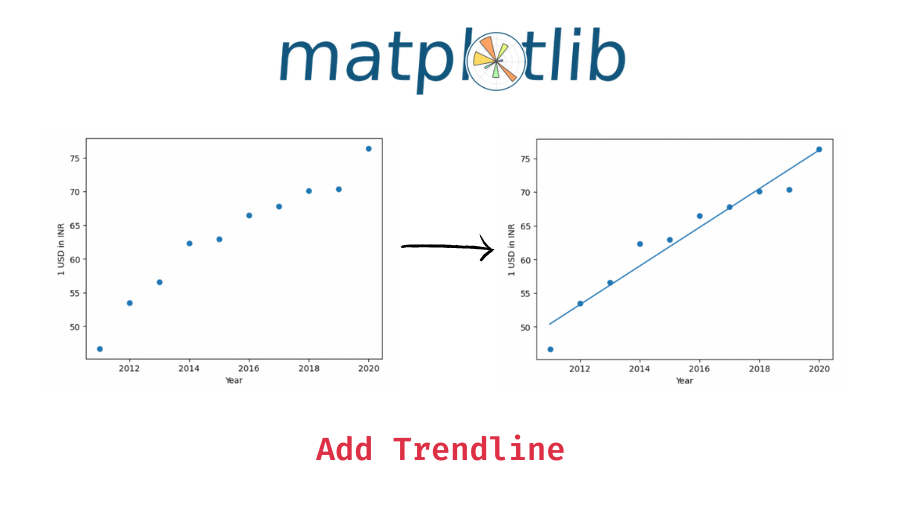# Add Trendline to a Maplotlib Plot with Code and Output

In this tutorial, we will look at how to add a trendline to a matplotlib scatter plot with the help of some examples.

## Steps to add a trendline to a plot

To add a trendline to a plot in matplotlib –

1. First, plot your scatter plot with the relevant points using the matplotlib pyplot’s `scatter()` function.
2. Create the trendline with the help of the `numpy.polyfit()` and the `numpy.plot1d()` functions.
3. Add the trendline to the matplotlib plot using the `matplotlib.pyplot.plot()` function.

The important part here is to get the trendline which we do with the help of the numpy functions `numpy.polyfit()` and `numpy.plot1d()`.

The `numpy.polyfit()` function is used to get a least squares polynomial fit. We pass the `x` and `y` values and the degree of the polynomial fit. For a line, use `1` as the degree of fit. The `numpy.polyfit()` function returns the coefficients of the polynomial which we then pass to the `numpy.plot1d()` function which creates a polynomial function from the given coefficients.

To get the trendline points, we use the resulting function from `numpy.plot1d()` and pass the x values to get the corresponding trendline points which we then plot on our matplotlib plot.

Let’s now look at an example of using the above steps.

## Example 1 – Add trendline to a plot

Let’s use the data of US dollar to Indian Rupee conversion to plot our scatter plot and get a trendline. We’ll use the conversion rates from 2011 to 2020.

```import numpy as np
import matplotlib.pyplot as plt

# x values - years
x = [2011, 2012, 2013, 2014, 2015, 2016, 2017, 2018, 2019, 2020]
# y values - 1 USD in INR
y = [46.67, 53.44, 56.57, 62.33, 62.97, 66.46, 67.79, 70.09, 70.39, 76.38]

# plot x and y on scatter plot
plt.scatter(x, y)
plt.xlabel('Year')
plt.ylabel('1 USD in INR')

# get the trendline coefficients
z = np.polyfit(x, y, 1)
# get the polynomial to generate the trendline
p = np.poly1d(z)

# add trendline to the plot
plt.plot(x, p(x))```

Output:

📚 Data Science Programs By Skill Level

Introductory

Intermediate ⭐⭐⭐

🔎 Find Data Science Programs 👨‍💻 111,889 already enrolled

Disclaimer: Data Science Parichay is reader supported. When you purchase a course through a link on this site, we may earn a small commission at no additional cost to you. Earned commissions help support this website and its team of writers.

Here, we first used the maplotlib pyplot’s `plt.scatter()` function to plot our scatter plot with the given x and y values. We then generated our trendline using the numpy `polyfit()` and `ploy1d()` functions and then used the `plt.plot()` function to add the trendline to our matplotlib plot.

## Example 2 – Add higher order trendcurve to the plot

In the above example, we added a trendline (a straight line) that best fits the scatter plot. You can similarly add fit curves with higher-degree polynomials to the plots.

The only change you need to make is to pass the degree you want the fit curve to have to the `numpy.polyfit()` function. For example, to add a quadratic fit curve use the degree as 2, to add a cubic fit curve, use the degree as 3, etc.

```# plot x and y on scatter plot
plt.scatter(x, y)
plt.xlabel('Year')
plt.ylabel('1 USD in INR')

# get the trendline coefficients
z = np.polyfit(x, y, 2)
# get the polynomial to generate the trendline
p = np.poly1d(z)

# add trendline to the plot
plt.plot(x, p(x))```

Output:

You can see that the fit line now is a quadratic curve and not a straight line.

Let’s add a cubic fit curve to the above points.

```# plot x and y on scatter plot
plt.scatter(x, y)
plt.xlabel('Year')
plt.ylabel('1 USD in INR')

# get the trendline coefficients
z = np.polyfit(x, y, 3)
# get the polynomial to generate the trendline
p = np.poly1d(z)

# add trendline to the plot
plt.plot(x, p(x))```

Output:

You can see that we get a fit curve on the plot.

You might also be interested in –

•